# Load Balancing and Parallel Computation Model for Performance and Accuracy over the Cluster of Nodes

Load Balancing and Parallel Computation Model for Performance and Accuracy over the Cluster of Nodes

Computer Science and Engineering, School of Technology, GITAM Deemed to be University, Hyderabad 502329, Telangana, India

Corresponding Author Email:
uma.15phani@gmail.com
Page:
343-348
|
DOI:
https://doi.org/10.18280/isi.270219
13 January 2022
|
Accepted:
19 April 2022
|
Published:
30 April 2022
| Citation

OPEN ACCESS

Abstract:

Keywords:

cloud computing, load balancing, parallel computing, service oriented architectures

1. Introduction

The load is a term commonly used in cloud computing to describe the feasibility of assigning different tasks to VMs. There are several ways to describe the load balancing issue.

(1) Allocation of Task- The arbitrary distribution of a limited number of tasks across multiple Physical Machines (PMs), each of which is assigned to a separate virtual machine (VM). How effective the job allocation to the cloud is what determines how effective the load balancing algorithm is [3-6].

(2) VM/Task Migration Management- Back in the Environment of Cloud Computing'' VM Migration is only the movements of the VM out of 1 PM to a different PM into advancing the reference use of this info centre where the PM continues to be overloaded. Likewise, the migration of this ongoing condition of some task in one VM to some other VM or VM of a single sponsor into VM of some other server is known as task migration. That really is why; both the VM or task migration plays an important part load balancing of cloud computing.

Communication and synchronization enable more efficient use of CPU resources in parallel processes. Overall, a data center's responsiveness to parallel tasks must be maintained while ensuring effective node utilization . However, load balancing is a significant problem in cloud computing, necessitating a distributed solution.

A major problem with cloud computing is the difficulty in properly balancing load by allocating tasks to the right servers and clients individually. It is therefore not cost-effective nor feasible to meet all the demand by keeping a few idle services running around. Even when tasks are allocated, there is still a degree of ambiguity .

ATLM/ADPM is thus proposed, with the goal of improving server throughput and performance, as well as maximizing the use of resources. The load balancing and parallel distributed computing drawbacks are solved within the existing protocols by using this protocol's cloud-based approach. In order to simplify the tasks and decrease the amount of time spent waiting and switching between them, as well as improve the computer's speed, as well as the server's throughput and efficiency.

2. Review Study

A method inspired by honey bee behavior was suggested in ref.  to create well balanced load among VMs in order to optimize throughput and balance the priority of activities on VMs. This technique is described in detail in ref. . Because of this, jobs in the queue don't have to spend a lot of time waiting. The average execution time and reduced waiting time for jobs in the queue were both improved as a result of using this method. This method is suitable for non-preemptive independent jobs in heterogeneous systems.

For cloud computing and queuing models for a collection of heterogeneous multi core servers of various sizes and speeds, performance optimization and power saving in data centers were addressed in ref. . For many heterogeneous multi core server processors, it tackles the issue of power allocation and load distribution across clouds and data centers, in particular. Even so, this is only a proof-of-concept research.

According to Rao et al. , the information nodes of all other nodes should be approached with a balanced manner. It's necessary for a node to query the other buttons when it gets work to see which one has less use to send it, and when all the nodes are querying overload occurs. The network will be burdened greatly if all nodes broadcast a status update. Time spent in performing query status at each node is the next problem to consider. Additionally, the present condition of the network has an impact on how well load balancing works. This is due to the fact that configuring a network node to find every other node in a complicated network with many subnets is a difficult job. As a result, checking the status of cloud nodes will have an impact on load balancing performance.

For example, reaction time has a significant impact on how well the cloud load balances performance. There were two problems with the previous method that were not addressed in this research. When the server is overloaded, load balancing takes place; otherwise, the computing cost and bandwidth usage rise. Authors have suggested an algorithm based on request response time to properly allocate necessary server choices, and this method has decreased query information on available resources, as well as contact with and computation on each of the servers individually .

However, the algorithm has not yet taken into account the burden of each resource, thus Min – Min  reduces the time it takes to perform the task in each network node. To address this shortcoming, the authors came up with the Load Balance Improved Min-Min (LBIMM) method. There will be many overloaded and idle resources if the workload of each resource is not taken into account. This has an impact on the load balancing of clouds. The present cloud computing scheduling method is based on the basic conventional Min-Min Algorithm.

Resource scheduling rules and resource load balancing in the cloud are important considerations for the LBRS (Load Balanced Resource Scheduling Method) algorithm developed by Kapur . Aims include increasing CPU utilization and throughput while lowering reaction times, ensuring that users do not have to wait, and adhering to the Fairness Principle. When discussing quality of service (QoS), the terms being used include throughput, response time, and waiting time. For load balancing, we use simulation and analysis of data on the effects of various factors. It was then that we found out how important the parameter of make span (runtime) is to the cloud data center infrastructure. As a result, the researchers' goal is to determine if the algorithms' load balancing is helpful in reducing virtual machines' make time.

The dynamic demand is spread over many resources via load balancing to ensure that no one resource is underused or overwhelmed, but this presents a significant optimization challenge. This article proposes a load balancing method based on Simulated Annealing (SA), with the main goal of balancing the cloud infrastructure's load. The efficacy of the algorithm is assessed using a customized version of a conventional Cloud Analyst simulator. The suggested method outperforms current approaches such as First Come First Serve (FCFS), local search algorithms such as Stochastic Hill Climbing (SHC), and Round Robin (RR) .

The user supplies the task requirements, including the job duration, and the scheduler uses those needs to make operational choices. Once an idle or least-laden VM is found, the load balancer uses the current status information to determine whether to move the job from the highly loaded one to the idle or least-loaded one. The resource monitor interacts with each VM's resource prober and gathers information about each VM's capabilities, current load, and number of tasks in the execution/waiting queue. The user supplies the task requirements, including the job duration, and the scheduler uses those needs to make operational choices.

A single computer gathers all the intermediate findings and reports them to a single machine that combines them all to get the final answers. This is how most parallelizable problems operate. As the software runs, it will identify which computer it is operating on and then attack the relevant portion of the original issue based on that knowledge. To receive results from all other computers once the calculation is complete, one machine will serve as a receiver. Each running program or process must be able to tell itself apart from other running programs for this method to function.

## 1.png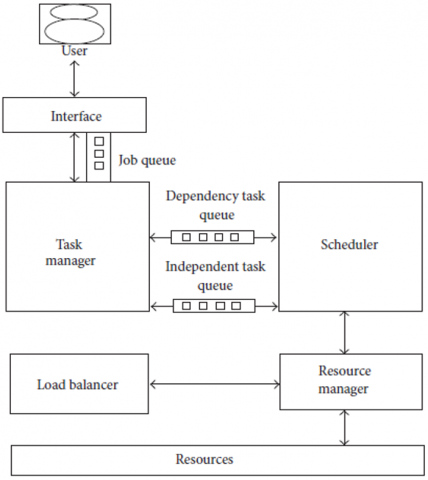Each of the VMs' capabilities is collected by the resource management by communicating with all of the VMs and obtaining the number of processor elements and the processing capacity of each of the processor elements. Using the processing capacity assigned to each VM, the resource management also determines the VM's weight. This also tells you how much RAM each of the virtual machines has set up for and is needed is shown in Figure 1. The load balancer determines the amount of tasks per VM and divides that number by the number of VMs. If the ratio is less than 1, the scheduler will be notified to choose a VM for the task; otherwise, the load on each VM will be calculated using the VMs' job execution list. If the utilization is less than 20%, the least used VM will be assigned; otherwise, the scheduler will be notified to determine the best VM for the task. A suitable VM will be found, and the Job will be assigned to it. The collection of computing resources is comprised of the configured data centers, which contain hosts and their VMs, as well as the associated processing components. In order to properly distribute task requests to a suitable resource, the resources are checked for idleness and high load.

A. Computation of Load balance factor

Represents the total load on all virtual machines,

$L=\sum_{i=1}^{k} l_{i}$,    (1)

where is the number of virtual machines in the data center? The following is an explanation of load per unit capacity:

$\mathrm{LPC}=\frac{L}{\sum_{i=1}^{m} C_{i}}$ Threshold $T_{i}=\mathrm{LPC}^{*} c_{i}$,    (2)

where, ci represents the node's overall capacity. The virtual machine's load imbalance factor is calculated as follows:

$If \quad V M\left\{\begin{array}{l}<\left|T_{i}-\sum_{v=1}^{k} L_{v}\right|, \text { Underloaded, } \\ >\left|T_{i}-\sum_{v=1}^{k} L_{v}\right|, \text { Overloaded, } \\ =\left|T_{i}-\sum_{i=1}^{k} l_{i}\right|, \text { Balanced. }\end{array}\right.$    (3)

Once the overloaded VM's load falls below a certain threshold, the migration of tasks from the overloaded VM to the under loaded VM may be permitted until the difference between the two values is i.

When the total load of all the VMs is less than the threshold value for that VM, it is considered under loaded. Under loaded VM takes load from overloaded VM till overloaded VM's load reaches threshold and difference is the λj.

The overloaded VM's load is transferred until it falls below the threshold. The under loaded VM can only take on load up to a certain point before it becomes overloaded.

B. Model Methodology

As a result of this approach, jobs and processing are allocated to VMs with the best processing capability based on information about each VM, such as its processing capacity, load on each VM, and the amount of time it will take to complete each work with its priority. This algorithm's static scheduling determines the proper VM allocation based on the processing capability of the VMs, the number of incoming tasks, and the duration of each job.

## 2.png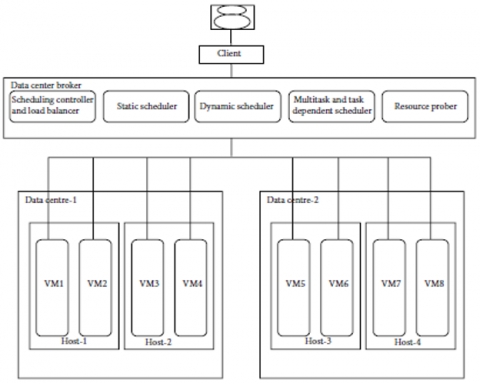Figure 2. Proposed architecture recent generation

C. Implementation Aspect of the Algorithm

Here, we propose a model built on the most recent generation of load balancing algorithms.

4. Model

Allowing for the existence of many virtual machines, let T=(T1, T2, T3, ..., Tn) be the list of tasks that each virtual machine should process. VMs run independently of one another, each using a different set of system resources as a result. Other VMs cannot access its resources. We allocate 'n' tasks to'm' VMs in a non-preemptive dependent schedule.

Processing Time. Let PTij be the processing time of assigning task ‘i’ to VM ‘j’ and define

$x_{i j}= \begin{cases}1, & \text { if task " } i \text { " is assigned } \\ 0, & \text { otherwise }\end{cases}$    (4)

Then the linear programming model is given as

Minimize $Z=\sum_{i=1}^{n} \sum_{j=1}^{m} \mathrm{PT}_{i j} x_{i j}$

Subjectto $: \sum_{i=1}^{n} x_{i j}=1, j=1,2, \ldots, m$

$x_{i j}=0 \text { or } 1 .$    (5)

Utilization of available resources. It's essential to maximize the use of resources, which comes from (6) and (7). It gets more difficult to achieve high levels of resource consumption. The new standard for determining average usage is [14-18].

Average utilization $=\frac{\sum_{j \in \mathrm{VMs}} C T_{j}}{\text { Makespan } * \text { Number of VMs }} \quad \quad \quad$,    (6)

where make span can be expressed as

Makespan $=\max \left\{\frac{C T_{j}}{j \in V M s}\right\} .$    (7)

Capacity of a VM. Consider

$C_{\mathrm{VM}}=\mathrm{pe}_{\mathrm{num}} * \mathrm{pe}_{\text {mips }}$,    (8)

where, CVM is the capacity of the VM (see (8)), penum is the number of processing elements in the VM, and penum is the million instructions per second of a PE.

Capacity of All the VMs. Consider

$C=\sum_{j=1}^{m} C_{\mathrm{VM}_{j}}$,    (9)

When all VMs are added together, the total capacity allocated to an application or environment is C.

$\mathrm{TL}=T_{\text {mips }} * T_{\text {pe }}$,    (10)

Job Length. Consider

$\mathrm{JL}=\sum_{k=1}^{p} \mathrm{TL}_{i}$,    (11)

where, 'P' seems to be the job's total number of interrelated tasks. Ratio of work to rest time. In (12) and (13) the task load ratio is computed to identify and distribute jobs to virtual machines. It may be summed up as

$\mathrm{TLR}_{i j}=\frac{\mathrm{TL}_{i}}{C_{\mathrm{VM}_{j}}}$,

$i=1,2, \ldots, n$ tasks $, j=1,2, \ldots, m$ Virtualmachines,    (12)

where, TLi is the task length which is estimated at the beginning of the execution and Cvm is the capacity of the VM. Consider

If $\mathrm{TLR}_{i j}= \begin{cases}0, & \text { assign the task to VM, } \\ \text { Otherwise, } & \text { Do not assign. }\end{cases}$    (13)

5. Results of Experiments and Analysis of Performance

The model's performance was examined using the CloudSim simulation results. To make use of the techniques, the CloudSim simulator's classes have been extended (overridden). As shown in the accompanying diagrams, resource circumstances affect everything from load balancing to response time to the number of job migrations data incorporated in Table 1.

Table 1. Load balancing and distributed processing in cloud computing environment using ADPM – ATLM

 S.No Entity Parameters Values 1 Task Center Length of task 5000-10000 Total number of task 50-250 File size 300-5000 2 Virtual Machine MIPS of pe 512-1024 Number of VM 10 Numberof peper vm 1-3 Bandwidth 500-1200 Memory 512-2055 Storage 100000-500000 Unit cost 1-10

In the cloud sim, set the same environmental parameters as the premise, we simulate the environment with round robin, weighted round robin and proposed ADPM - ATLM models. In the aspects of task management over the load balancing and distributed processing on the work loads.

## 3.png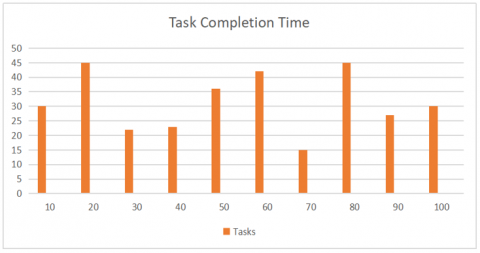Figure 3. Displays the number of tasks allocated

The figure displays the number of tasks allocated for each and every virtual machine, the task is randomly distributed without any load balancing measures.

Comparison of the load balancing methods with different models in shows Figure 4.

Task execution on the load balanced virtual machines shown in Figure 5, In order to perform which leads the migration of the jobs from one VM to other VM as to not over load the VM, in the execution time shows in Figure 6.

## 4.png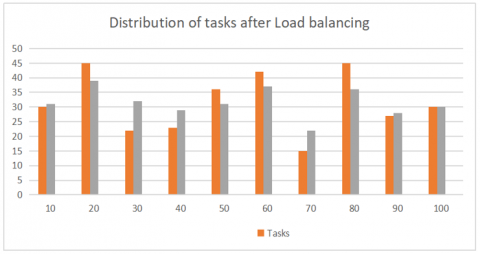Figure 4. Distribution of the task after performing the load balancing proposed methods

## 5.png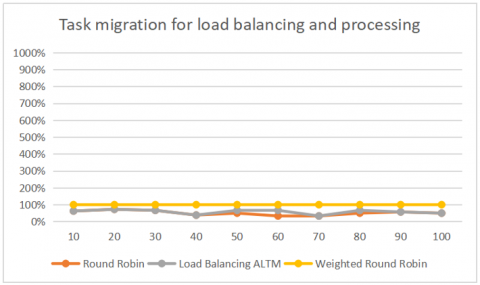## 6.png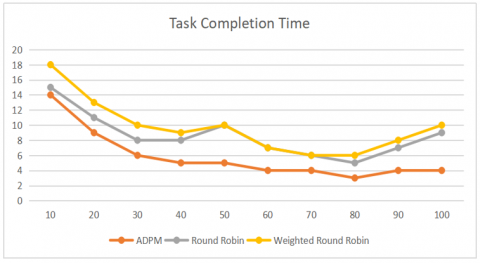The parallel distributed processing has been implemented on the task distributed over the VMs and the model outward performs in a best way in terms of efficiency.

6. Conclusion

In this work, the improved models have the capabilities of load balancing on each VM and identifying the task length in order to perform the distributed processing over the VM. This section will apply the load balancing model even for the task execution as to shift the task from one Vm to other in terms of executing the task in a better way. The enhanced model's load balancer runs after each job has been completed. Because the loads are equally spread and handled across all VMs, there is no idle time in the participating resources at the conclusion of each job (VMs).

References

 Bohn, R.B., Messina, J., Liu, F., Tong, J., Mao, J. (2011). NIST cloud computing reference architecture. In 2011 IEEE World Congress on Services, pp. 594-596. https://doi.org/10.1109/SERVICES.2011.105

 Tsai, C.W., Rodrigues, J.J. (2013). Metaheuristic scheduling for cloud: A survey. IEEE Systems Journal, 8(1): 279-291. https://doi.org/10.1109/JSYST.2013.2256731

 Mishra, S.K., Puthal, D., Sahoo, B., Jena, S.K., Obaidat, M.S. (2018). An adaptive task allocation technique for green cloud computing. The Journal of Supercomputing, 74(1): 370-385. https://doi.org/10.1007/s11227-017-2133-4

 Volkova, V.N., Chemenkaya, L.V., Desyatirikova, E.N., Hajali, M., Khodar, A., Osama, A. (2018). Load balancing in cloud computing. In 2018 IEEE Conference of Russian Young Researchers in Electrical and Electronic Engineering (EIConRus), pp. 387-390. https://doi.org/10.1109/EIConRus.2018.8317113

 Parida, S., Panchal, B. (2018). An efficient dynamic load balancing algorithm using machine learning technique in cloud environment. International Journal of Scientific Research in Science, Engineering and Technology, 4(4): 1184-1186.

 Shah, J.M., Kotecha, K., Pandya, S., Choksi, D.B., Joshi, N. (2017). Load balancing in cloud computing: Methodological survey on different types of algorithm. In 2017 International Conference on Trends in Electronics and Informatics (ICEI), pp. 100-107. https://doi.org/10.1109/ICOEI.2017.8300865

 LD, D.B., Krishna, P.V. (2013). Honey bee behavior inspired load balancing of tasks in cloud computing environments. Applied Soft Computing, 13(5): 2292-2303. https://doi.org/10.1016/j.asoc.2013.01.025

 Cao, J., Li, K., Stojmenovic, I. (2013). Optimal power allocation and load distribution for multiple heterogeneous multicore server processors across clouds and data centers. IEEE Transactions on Computers, 63(1): 45-58. https://doi.org/10.1109/TC.2013.122

 Rao, P.S., Rao, V.P.C., Govardhan, A. (2013). Dynamic load balancing with central monitoring of distributed job processing system. International Journal of Computer Applications, 65(21): 43-47.

 Sharma, A., Peddoju, S.K. (2014). Response time based load balancing in cloud computing. In 2014 International Conference on Control, Instrumentation, Communication and Computational Technologies (ICCICCT), pp. 1287-1293. https://doi.org/10.1109/ICCICCT.2014.6993159

 Chen, H., Wang, F., Helian, N., Akanmu, G. (2013). User-priority guided Min-Min scheduling algorithm for load balancing in cloud computing. In 2013 National Conference on Parallel Computing Technologies (PARCOMPTECH), pp. 1-8. https://doi.org/10.1109/ParCompTech.2013.6621389

 Kapur, R. (2015). A workload balanced approach for resource scheduling in cloud computing. In 2015 eighth international conference on contemporary computing (IC3), pp. 36-41. https://doi.org/10.1109/IC3.2015.7346649

 Mondal, B., Choudhury, A. (2015). Simulated annealing (SA) based load balancing strategy for cloud computing. International Journal of Computer Science and Information Technologies, 6(4): 3307-3312.

 Kushwaha, M., Gupta, S. (2015). Response time reduction and performance analysis of load balancing algorithms at peak hours in cloud computing. International Journal of Computer Applications, 128(17): 26-31.

 Sharma, S., Luhach, A.K., Abdhullah, S.S. (2016). An optimal load balancing strategy for virtual machine in cloud environment. International Journal of Computer Science and Engineering, 9(28): 1-4. https://doi.org/10.17485/ijst/2016/v9i28/98384

 Prasad, V. (2014). Load balancing and scheduling of tasks in parallel processing environment. Int. J. Inf. Comput. Technol, 4(16): 1727-1732.

 Srivastava, S., Dadheech, P., Beniwal, M.K. (2011). Load balancing using high performance computing cluster programming. International Journal of Computer Science Issues (IJCSI), 8(1): 62-65.

 Afzal, S., Kavitha, G. (2019). Load balancing in cloud computing–A hierarchical taxonomical classification. Journal of Cloud Computing, 8(1): 1-24. https://doi.org/10.1186/s13677-019-0146-7